Documentation

# step

Step response plot of dynamic system; step response data

## Syntax

```step(sys) step(sys,Tfinal) step(sys,t) step(sys1,sys2,...,sysN) step(sys1,sys2,...,sysN,Tfinal) step(sys1,sys2,...,sysN,t) y = step(sys,t) [y,t] = step(sys) [y,t] = step(sys,Tfinal) [y,t,x] = step(sys) [y,t,x,ysd] = step(sys) [y,...] = step(sys,...,options) ```

## Description

`step` calculates the step response of a dynamic system. For the state-space case, zero initial state is assumed. When it is invoked with no output arguments, this function plots the step response on the screen.

`step(sys) ` plots the step response of an arbitrary dynamic system model, `sys`. This model can be continuous- or discrete-time, and SISO or MIMO. The step response of multi-input systems is the collection of step responses for each input channel. The duration of simulation is determined automatically, based on the system poles and zeros.

`step(sys,Tfinal)` simulates the step response from `t = 0` to the final time `t = Tfinal`. Express `Tfinal` in the system time units, specified in the `TimeUnit` property of `sys`. For discrete-time systems with unspecified sample time (```Ts = -1```), `step` interprets `Tfinal` as the number of sampling periods to simulate.

`step(sys,t)` uses the user-supplied time vector `t` for simulation. Express `t` in the system time units, specified in the `TimeUnit` property of `sys`. For discrete-time models, `t` should be of the form `Ti:Ts:Tf`, where `Ts` is the sample time. For continuous-time models, `t` should be of the form `Ti:dt:Tf`, where `dt` becomes the sample time of a discrete approximation to the continuous system (see Algorithms). The `step` command always applies the step input at `t=0`, regardless of `Ti`.

To plot the step response of several models `sys1`,..., `sysN` on a single figure, use

`step(sys1,sys2,...,sysN)`

`step(sys1,sys2,...,sysN,Tfinal)`

`step(sys1,sys2,...,sysN,t)`

All of the systems plotted on a single plot must have the same number of inputs and outputs. You can, however, plot a mix of continuous- and discrete-time systems on a single plot. This syntax is useful to compare the step responses of multiple systems.

You can also specify a distinctive color, linestyle, marker, or all three for each system. For example,

```step(sys1,'y:',sys2,'g--') ```

plots the step response of `sys1` with a dotted yellow line and the step response of `sys2` with a green dashed line.

When invoked with output arguments:

`y = step(sys,t)`

`[y,t] = step(sys)`

`[y,t] = step(sys,Tfinal)`

`[y,t,x] = step(sys) `

`step` returns the output response `y`, the time vector `t` used for simulation (if not supplied as an input argument), and the state trajectories `x` (for state-space models only). No plot generates on the screen. For single-input systems, `y` has as many rows as time samples (length of `t`), and as many columns as outputs. In the multi-input case, the step responses of each input channel are stacked up along the third dimension of `y`. The dimensions of `y` are then

`$\left(length\text{\hspace{0.17em}}of\text{\hspace{0.17em}}t\right)×\left(number\text{\hspace{0.17em}}of\text{\hspace{0.17em}}outputs\right)×\left(number\text{\hspace{0.17em}}of\text{\hspace{0.17em}}inputs\right)$`

and `y(:,:,j)` gives the response to a unit step command injected in the `j`th input channel. Similarly, the dimensions of `x` are

`$\left(length\text{\hspace{0.17em}}of\text{\hspace{0.17em}}t\right)×\left(number\text{\hspace{0.17em}}of\text{\hspace{0.17em}}states\right)×\left(number\text{\hspace{0.17em}}of\text{\hspace{0.17em}}inputs\right)$`

For identified models (see `idlti` and `idnlmodlel`) ```[y,t,x,ysd] = step(sys) ``` also computes the standard deviation `ysd` of the response y (`ysd` is empty if `sys` does not contain parameter covariance information).

`[y,...] = step(sys,...,options)` specifies additional options for computing the step response, such as the step amplitude or input offset. Use `stepDataOptions` to create the option set `options`.

## Examples

collapse all

Plot the step response of the following second-order state-space model:

`$\begin{array}{l}\left[\begin{array}{c}{\underset{}{\overset{˙}{x}}}_{1}\\ {\underset{}{\overset{˙}{x}}}_{2}\end{array}\right]=\left[\begin{array}{cc}-0.5572& -0.7814\\ 0.7814& 0\end{array}\right]\left[\begin{array}{c}{x}_{1}\\ {x}_{2}\end{array}\right]+\left[\begin{array}{cc}1& -1\\ 0& 2\end{array}\right]\left[\begin{array}{c}{u}_{1}\\ {u}_{2}\end{array}\right]\\ y=\left[\begin{array}{cc}1.9691& 6.4493\end{array}\right]\left[\begin{array}{c}{x}_{1}\\ {x}_{2}\end{array}\right]\end{array}$`

```a = [-0.5572,-0.7814;0.7814,0]; b = [1,-1;0,2]; c = [1.9691,6.4493]; sys = ss(a,b,c,0); step(sys)```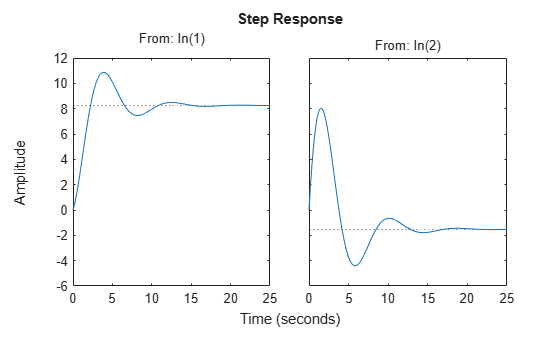The left plot shows the step response of the first input channel, and the right plot shows the step response of the second input channel.

Create a feedback loop with delay and plot its step response.

```s = tf('s'); G = exp(-s) * (0.8*s^2+s+2)/(s^2+s); T = feedback(ss(G),1); step(T)```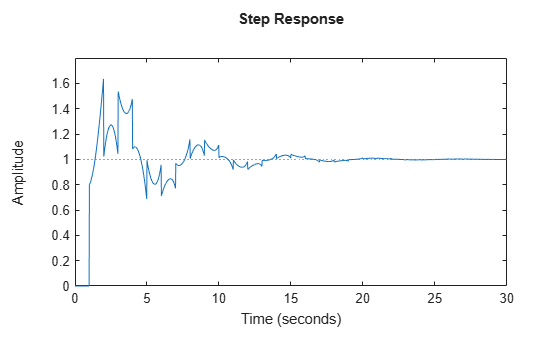The system step response displayed is chaotic. The step response of systems with internal delays may exhibit odd behavior, such as recurring jumps. Such behavior is a feature of the system and not software anomalies.

Compare the step response of a parametric identified model to a non-parametric (empirical) model. Also view their 3 $\sigma$ confidence regions.

`load iddata1 z1`

Estimate a parametric model.

`sys1 = ssest(z1,4);`

Estimate a non-parametric model.

`sys2 = impulseest(z1);`

Plot the step responses for comparison.

```t = (0:0.1:10)'; [y1, ~, ~, ysd1] = step(sys1,t); [y2, ~, ~, ysd2] = step(sys2,t); plot(t, y1, 'b', t, y1+3*ysd1, 'b:', t, y1-3*ysd1, 'b:') hold on plot(t, y2, 'g', t, y2+3*ysd2, 'g:', t, y2-3*ysd2, 'g:')```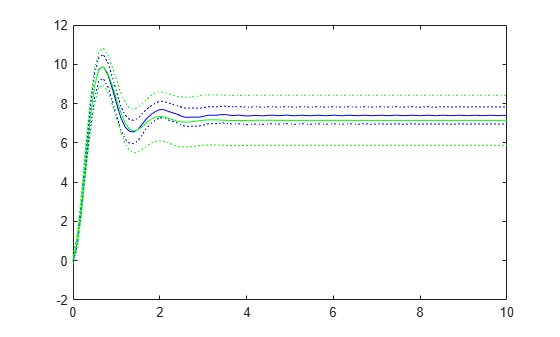Validate the linearization of a nonlinear ARX model by comparing the small amplitude step responses of the linear and nonlinear models.

`load iddata2 z2;`

Estimate a nonlinear ARX model.

`nlsys = nlarx(z2,[4 3 10],'tree','custom',{'sin(y1(t-2)*u1(t))+y1(t-2)*u1(t)+u1(t).*u1(t-13)','y1(t-5)*y1(t-5)*y1(t-1)'},'nlr',[1:5, 7 9]);`

Determine an equilibrium operating point for `nlsys` corresponding to a steady-state input value of 1.

```u0 = 1; [X,~,r] = findop(nlsys, 'steady', 1); y0 = r.SignalLevels.Output;```

Obtain a linear approximation of `nlsys` at this operating point.

`sys = linearize(nlsys,u0,X);`

Validate the usefulness of `sys` by comparing its small-amplitude step response to that of `nlsys`.

The nonlinear system `nlsys` is operating at an equilibrium level dictated by `(u0, y0)`. Introduce a step perturbation of size 0.1 about this steady-state and compute the corresponding response.

```opt = stepDataOptions; opt.InputOffset = u0; opt.StepAmplitude = 0.1; t = (0:0.1:10)'; ynl = step(nlsys, t, opt);```

The linear system `sys` expresses the relationship between the perturbations in input to the corresponding perturbation in output. It is unaware of the nonlinear system's equilibrium values.

Plot the step response of the linear system.

```opt = stepDataOptions; opt.StepAmplitude = 0.1; yl = step(sys, t, opt);```

Add the steady-state offset, `y0` , to the response of the linear system and plot the responses.

```plot(t, ynl, t, yl+y0) legend('Nonlinear', 'Linear with offset')```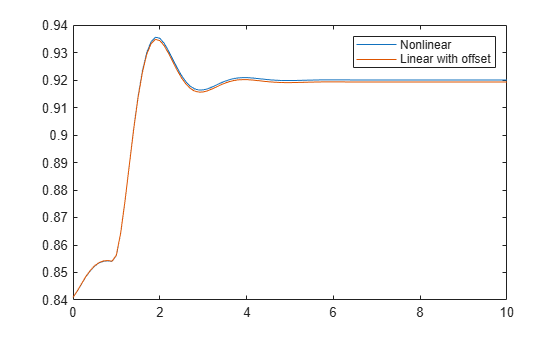Compute the step response of an identified time-series model.

A time-series model, also called a signal model, is one without measured input signals. The step plot of this model uses its (unmeasured) noise channel as the input channel to which the step signal is applied.

`load iddata9;`

Estimate a time-series model.

`sys = ar(z9, 4);`

`ys` is a model of the form `A y(t) = e(t)` , where `e(t)` represents the noise channel. For computation of step response, `e(t)` is treated as an input channel, and is named `e@y1`.

Plot the step response.

`step(sys)`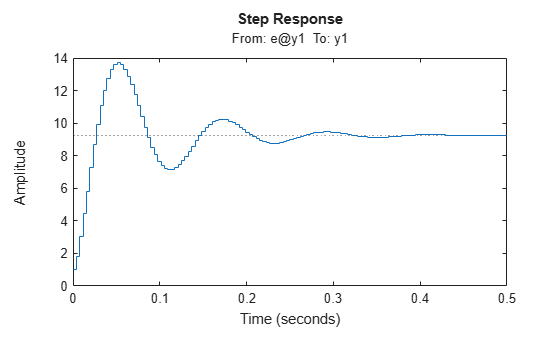## Tips

You can change the properties of your plot, for example the units. For information on the ways to change properties of your plots, see Ways to Customize Plots (Control System Toolbox).

## Algorithms

Continuous-time models without internal delays are converted to state space and discretized using zero-order hold on the inputs. The sample time is chosen automatically based on the system dynamics, except when a time vector `t = 0:dt:Tf` is supplied (`dt` is then used as sampling period). The resulting simulation time steps `t` are equisampled with spacing `dt`.

For systems with internal delays, Control System Toolbox™ software uses variable step solvers. As a result, the time steps `t` are not equisampled.

## References

 L.F. Shampine and P. Gahinet, "Delay-differential-algebraic equations in control theory," Applied Numerical Mathematics, Vol. 56, Issues 3–4, pp. 574–588.